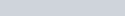ChemTalk

# Raoult’s Law EquationRaoult’s law is used to determine the vapor pressure of ideal solutions with nonvolatile solutes based on the vapor pressure of the solvent and the mole fraction of the solvent. Below we discuss what the equation of Raoult’s law is, dive into an explanation of how the law works, and share some example problems.

## Raoult’s Law Equation

The equation for Raoult’s law for nonvolatile solutes is below: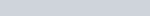•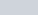is the vapor pressure of the solution.
•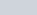is the vapor pressure of the solvent at the same temperature as the solution is measured.
•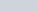is the mole fraction of the solvent in the solution.

## Raoult’s Law Explanation

Raoult’s law is used with solutions with nonvolatile solutes to determine the vapor pressure of the solution. The vapor pressure of the solution depends on the vapor pressure of the pure solvent and the mole fraction of the solvent in the solution.

As the amount of solute in the solution increases, the vapor pressure of the solution will decrease. The absolute value of the vapor pressure will depend on the original vapor pressure of the solvent.

This formula works best for ideal solutions and non-volatile solutes. A non-volatile solute does not tend to evaporate. For example, salt in saltwater. When the water evaporates it leaves behind the salt.

For a refresher on how to determine mole fraction, see this article. In simple terms, the mole fraction of solvent is the moles of solvent divided by the total moles in solution.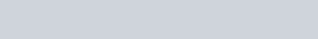## Limitations of Raoult’s Law

Raoult’s law does not work perfectly in all situations. Below are the two main limitations of the law.

Ideal Solution – The solution must be an ideal solution for Raoult’s law to apply. An ideal solution is not very concentrated or very very dilute. There should also be similar intermolecular forces between solute and solvent as there were between just solvent molecules for an ideal solution.

Moles of Solute – It must be considered if the solute dissociates in solution. For example, if NaCl (salt) is added to water it will dissociate into Na+ and Cl. These will then act as twice as many moles of solute as the moles of NaCl added. This should be taken into account.

## Why Does this Effect Occur?

The decreased vapor pressure with increasing solute concentration is due to the molecules on the surface. When it is pure solvent, all the molecules on the surface are solvent molecules. These surface molecules are also the ones that will dissociate into the vapor phase. As the solute concentration increases, there are fewer solvent molecules on the surface to escape to the vapor phase. Some of those surface spots are now occupied by solute molecules. This decreases the overall vapor pressure.On the left there is no solute in solution and all the surface spots are occupied by solvent molecules (purple). When solute (orange) is added to the solution (right) there are now fewer solvent molecules on the surface because solute molecules occupy some of the spots.

## Example Problem

Example Problem:

Consider a solution of ethanol at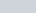. You add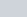of sucrose (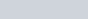) to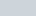of ethanol (). The original vapor pressure for ethanol was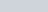.  What is the new vapor pressure of the ethanol and sucrose solution at?

Worked Example Solution:

In this example our solvent is ethanol and our solute is sucrose. Based on Raoult’s equation, to determine the new vapor pressure we need to know the original vapor pressure of the solvent and the new mole fraction of the solvent.The question tells us the original vapor pressure,, is. The only other variable we need is the mole fraction of solvent,.

To find the mole fraction of solvent we first need to convert our grams of sucrose and our grams of ethanol to moles.Then we can solve for the mole fraction of solvent: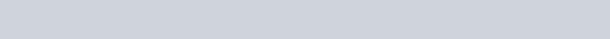Now we have all the variables we need and can plug them into Raoult’s law equation.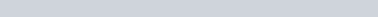The final answer comes out to be a vapor pressure of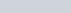. The pressure lowers by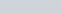due to the solute in the solution.

## Experimental Ways to Observe Raoult’s Law

There are several experiments you can do to observe Raoult’s law in action!

First, you could compare the evaporation rate of a beaker of a pure solvent to a beaker of the solution made of the same solvent. Make sure both beakers have the same volume of the overall solution. After being allowed to sit for a day or so, there will be a noticeable difference in volume between the two. The pure solvent will evaporate faster because it has a higher vapor pressure.

A second way to observe Raoult’s law is to use a manometer. A manometer is a closed container that connects to a U-shaped tube with mercury in it. The other end of the tube is open to the atmosphere. Using the manometer, the vapor pressure of both the pure solvent and the solution could be measured.

## Raoult’s Law Practice Problems

Problem 1

Imagine an unknown solvent with an unknown mix of solutes. Then, imagine a chemcial reaction occurs inside the solvent which decreases the total moles of solute as well as release heat to raise the temperature of the solvent. Based on Raoult’s Law and the Ideal Gas Law, would you expect the solvent’s vapor pressure to increase or decrease?

Problem 2

At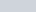an unknown solvent has a vapor pressure of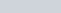, when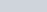of solvent contains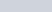of unkown solvent. Calculate the vapor pressure of the unknown solvent when pure at.

## Raoult’s Law Practice Problem Solutions

1: Vapor pressure would increase

2: